# 0x00 背景

Rtl-sdr:原身就是Realtek RTL2832U（瑞昱的一款电视棒）。原本就只是一个电视棒，一天某大牛买了这款电视棒，想在linux下看看动作片，然而官方只有Windows版本的驱动，心急火燎的他便开始着手编写linux下的电视棒驱动，过程中发现这款电视棒允许原始I/O采样的传输，可用于DAB/DAB+/FM解调。于是他拉起裤子，开始了进一步的研究…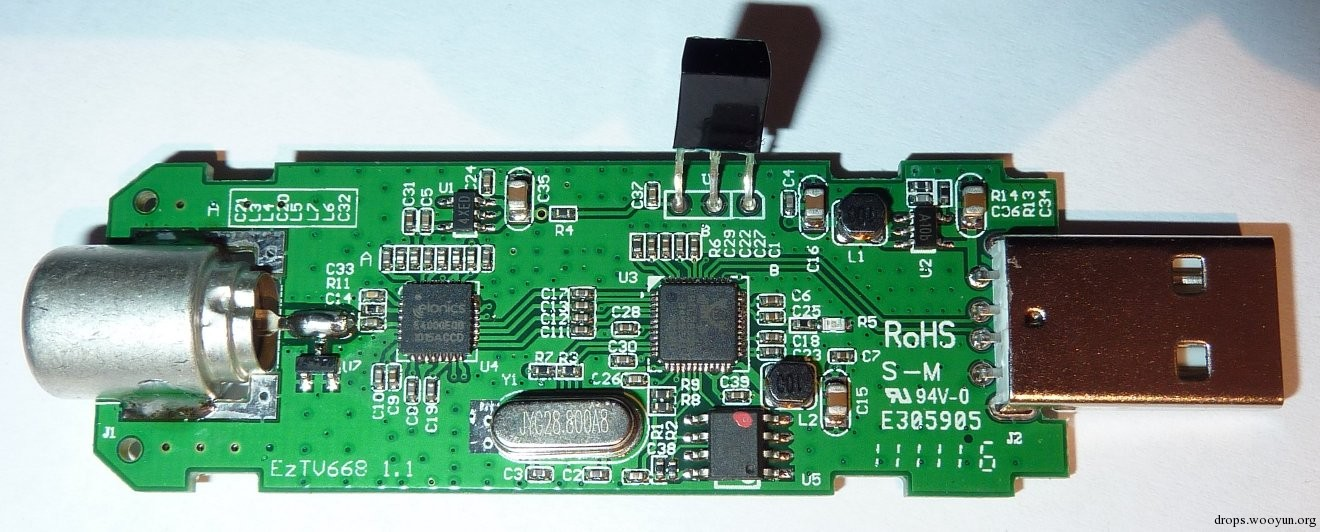Wireshark（数据包监听，查看，大家都很熟悉）

Airprobe(GSM信号接受和解密)

GSM网络默认使用A5/1加密算法。如果要得到原始的数据，需要将截获的数据进行破解，一般是用一个大约2T的“彩虹表”进行碰撞。但国内GMS网络据说没有加密。

Kali首先apt-get update 不用多说。但是运行这条命令之前最好检查一下你的

sources.list(/etc/apt/sources.list)文件里有这两条：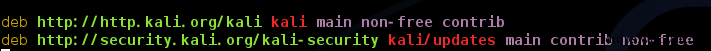Linux系其他用户可以执行一下脚本安装：

``````#!bash
apt-get install cmake
apt-get install libusb-1.0.0-dev
apt-get install libpulse-dev
apt-get install libboost-all-dev

git clone git://git.osmocom.org/rtl-sdr.git
git clone git://git.osmocom.org/osmo-sdr
git clone git://git.osmocom.org/gr-osmosdr
git clone git://git.osmocom.org/csete/gqrx.git

mkdir sdr
cd sdr

``````

# 0x02 安装Airprobe

## 1. 各种依赖包依赖库：（少装一个都不行！）

``````#!bash
sudo apt-get install git-core autoconf automake libtool g++ python-dev swig libpcap0.8-dev cmake git libboost-all-dev libusb-1.0-0 libusb-1.0-0-dev libfftw3-dev swig python-numpy libpulse-dev libpcsclite-dev
``````

``````#!bash
[email protected]/* <![CDATA[ */!function(t,e,r,n,c,a,p){try{t=document.currentScript||function(){for(t=document.getElementsByTagName('script'),e=t.length;e--;)if(t[e].getAttribute('data-cfhash'))return t[e]}();if(t&#038;&#038;(c=t.previousSibling)){p=t.parentNode;if(a=c.getAttribute('data-cfemail')){for(e='',r='0x'+a.substr(0,2)|0,n=2;a.length-n;n+=2)e+='%'+('0'+('0x'+a.substr(n,2)^r).toString(16)).slice(-2);p.replaceChild(document.createTextNode(decodeURIComponent(e)),c)}p.removeChild(t)}}catch(u){}}()/* ]]&gt; */:~# cd /opt/
[email protected]/* <![CDATA[ */!function(t,e,r,n,c,a,p){try{t=document.currentScript||function(){for(t=document.getElementsByTagName('script'),e=t.length;e--;)if(t[e].getAttribute('data-cfhash'))return t[e]}();if(t&#038;&#038;(c=t.previousSibling)){p=t.parentNode;if(a=c.getAttribute('data-cfemail')){for(e='',r='0x'+a.substr(0,2)|0,n=2;a.length-n;n+=2)e+='%'+('0'+('0x'+a.substr(n,2)^r).toString(16)).slice(-2);p.replaceChild(document.createTextNode(decodeURIComponent(e)),c)}p.removeChild(t)}}catch(u){}}()/* ]]&gt; */:/opt# mkdir gsm
[email protected]/* <![CDATA[ */!function(t,e,r,n,c,a,p){try{t=document.currentScript||function(){for(t=document.getElementsByTagName('script'),e=t.length;e--;)if(t[e].getAttribute('data-cfhash'))return t[e]}();if(t&#038;&#038;(c=t.previousSibling)){p=t.parentNode;if(a=c.getAttribute('data-cfemail')){for(e='',r='0x'+a.substr(0,2)|0,n=2;a.length-n;n+=2)e+='%'+('0'+('0x'+a.substr(n,2)^r).toString(16)).slice(-2);p.replaceChild(document.createTextNode(decodeURIComponent(e)),c)}p.removeChild(t)}}catch(u){}}()/* ]]&gt; */:/opt# cd gsm/
``````

## 2. 安装libosmocore

``````#!bash
[email protected]/* <![CDATA[ */!function(t,e,r,n,c,a,p){try{t=document.currentScript||function(){for(t=document.getElementsByTagName('script'),e=t.length;e--;)if(t[e].getAttribute('data-cfhash'))return t[e]}();if(t&#038;&#038;(c=t.previousSibling)){p=t.parentNode;if(a=c.getAttribute('data-cfemail')){for(e='',r='0x'+a.substr(0,2)|0,n=2;a.length-n;n+=2)e+='%'+('0'+('0x'+a.substr(n,2)^r).toString(16)).slice(-2);p.replaceChild(document.createTextNode(decodeURIComponent(e)),c)}p.removeChild(t)}}catch(u){}}()/* ]]&gt; */:/opt/gsm# git clone git://git.osmocom.org/libosmocore.git
``````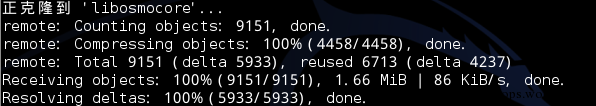## 3. 安装airprobe

``````#!bash
[email protected]/* <![CDATA[ */!function(t,e,r,n,c,a,p){try{t=document.currentScript||function(){for(t=document.getElementsByTagName('script'),e=t.length;e--;)if(t[e].getAttribute('data-cfhash'))return t[e]}();if(t&#038;&#038;(c=t.previousSibling)){p=t.parentNode;if(a=c.getAttribute('data-cfemail')){for(e='',r='0x'+a.substr(0,2)|0,n=2;a.length-n;n+=2)e+='%'+('0'+('0x'+a.substr(n,2)^r).toString(16)).slice(-2);p.replaceChild(document.createTextNode(decodeURIComponent(e)),c)}p.removeChild(t)}}catch(u){}}()/* ]]&gt; */:/opt/gsm# git clone git://svn.berlin.ccc.de/airprobe
``````

``````#!bash
./bootstrap
./configure
make
``````

# 0x03 START TO HACK

ppm offset或frequency offset：频率偏移，俗称偏频，一般由于硬件信号的源宿时钟不同步造成。

``````#!bash
[email protected]/* <![CDATA[ */!function(t,e,r,n,c,a,p){try{t=document.currentScript||function(){for(t=document.getElementsByTagName('script'),e=t.length;e--;)if(t[e].getAttribute('data-cfhash'))return t[e]}();if(t&#038;&#038;(c=t.previousSibling)){p=t.parentNode;if(a=c.getAttribute('data-cfemail')){for(e='',r='0x'+a.substr(0,2)|0,n=2;a.length-n;n+=2)e+='%'+('0'+('0x'+a.substr(n,2)^r).toString(16)).slice(-2);p.replaceChild(document.createTextNode(decodeURIComponent(e)),c)}p.removeChild(t)}}catch(u){}}()/* ]]&gt; */:~# kal -h
kalibrate v0.4.1-rtl, Copyright (c) 2010, Joshua Lackey
modified for use with rtl-sdr devices, Copyright (c) 2012, Steve Markgraf
Usage:
GSM Base Station Scan:
kal <-s band indicator> [options]

Clock Offset Calculation:
kal <-f frequency | -c channel> [options]

Where options are:
-s  band to scan (GSM850, GSM-R, GSM900, EGSM, DCS, PCS)
-f  frequency of nearby GSM base station
-c  channel of nearby GSM base station
-b  band indicator (GSM850, GSM-R, GSM900, EGSM, DCS, PCS)
-g  gain in dB
-d  rtl-sdr device index
-e  initial frequency error in ppm
-v  verbose
-D  enable debug messages
-h  help
``````

``````#!bash
[email protected]/* <![CDATA[ */!function(t,e,r,n,c,a,p){try{t=document.currentScript||function(){for(t=document.getElementsByTagName('script'),e=t.length;e--;)if(t[e].getAttribute('data-cfhash'))return t[e]}();if(t&#038;&#038;(c=t.previousSibling)){p=t.parentNode;if(a=c.getAttribute('data-cfemail')){for(e='',r='0x'+a.substr(0,2)|0,n=2;a.length-n;n+=2)e+='%'+('0'+('0x'+a.substr(n,2)^r).toString(16)).slice(-2);p.replaceChild(document.createTextNode(decodeURIComponent(e)),c)}p.removeChild(t)}}catch(u){}}()/* ]]&gt; */:~# kal -s 900
``````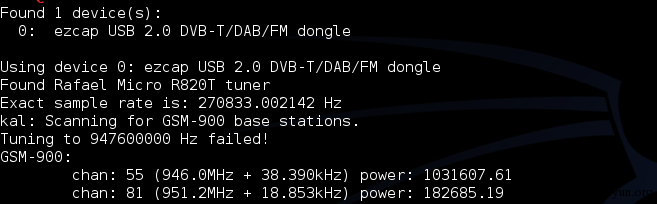``````#!bash
[email protected]/* <![CDATA[ */!function(t,e,r,n,c,a,p){try{t=document.currentScript||function(){for(t=document.getElementsByTagName('script'),e=t.length;e--;)if(t[e].getAttribute('data-cfhash'))return t[e]}();if(t&#038;&#038;(c=t.previousSibling)){p=t.parentNode;if(a=c.getAttribute('data-cfemail')){for(e='',r='0x'+a.substr(0,2)|0,n=2;a.length-n;n+=2)e+='%'+('0'+('0x'+a.substr(n,2)^r).toString(16)).slice(-2);p.replaceChild(document.createTextNode(decodeURIComponent(e)),c)}p.removeChild(t)}}catch(u){}}()/* ]]&gt; */:~# kal -c 55
``````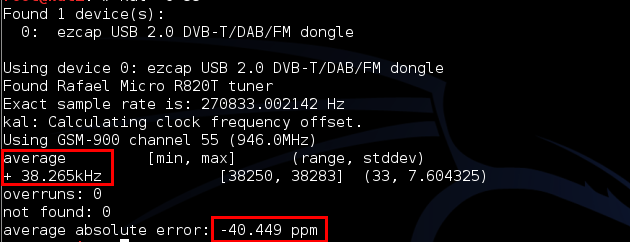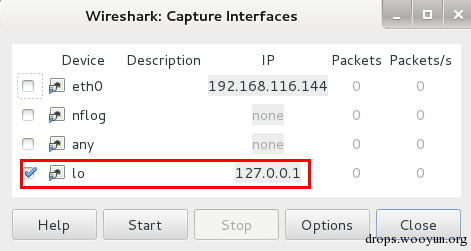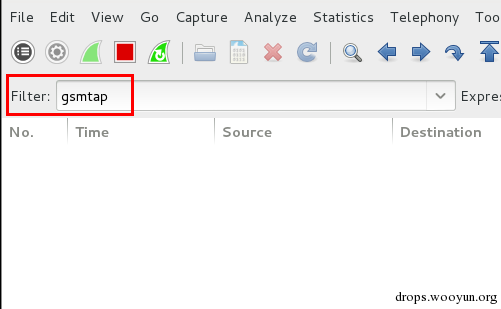``````#!bash
[email protected]/* <![CDATA[ */!function(t,e,r,n,c,a,p){try{t=document.currentScript||function(){for(t=document.getElementsByTagName('script'),e=t.length;e--;)if(t[e].getAttribute('data-cfhash'))return t[e]}();if(t&#038;&#038;(c=t.previousSibling)){p=t.parentNode;if(a=c.getAttribute('data-cfemail')){for(e='',r='0x'+a.substr(0,2)|0,n=2;a.length-n;n+=2)e+='%'+('0'+('0x'+a.substr(n,2)^r).toString(16)).slice(-2);p.replaceChild(document.createTextNode(decodeURIComponent(e)),c)}p.removeChild(t)}}catch(u){}}()/* ]]&gt; */:/opt/gsm/airprobe/gsm-receiver/src/python# ./gsm_receive_rtl.py -s 1e6 -f 946M
``````

Airprobe支持的控制类型：

``````#!bash
0C : TimeSlot0  "Combined configuration", with SDCCH/4
(FCCH + SCH + BCCH + CCCH + SDCCH/4)
0B : TS0  "FCCH + SCH + BCCH + CCCH"
1S : TS1  SDCCH/8
2T : TS2  (Full Rate) Traffic
1TE: TS1  Enhanced Full Rate Traffic
``````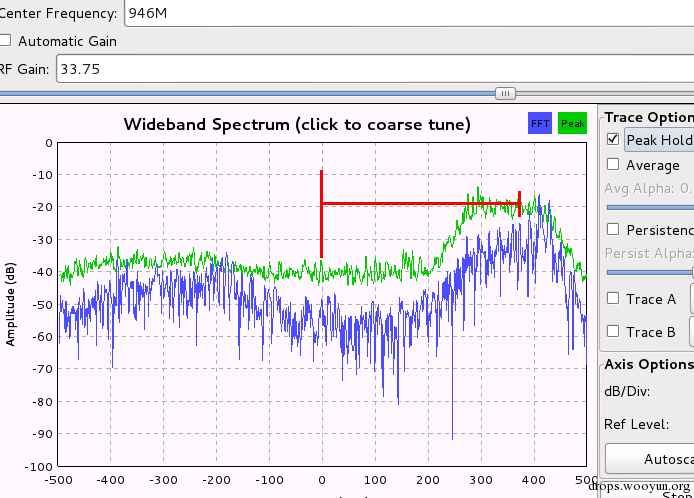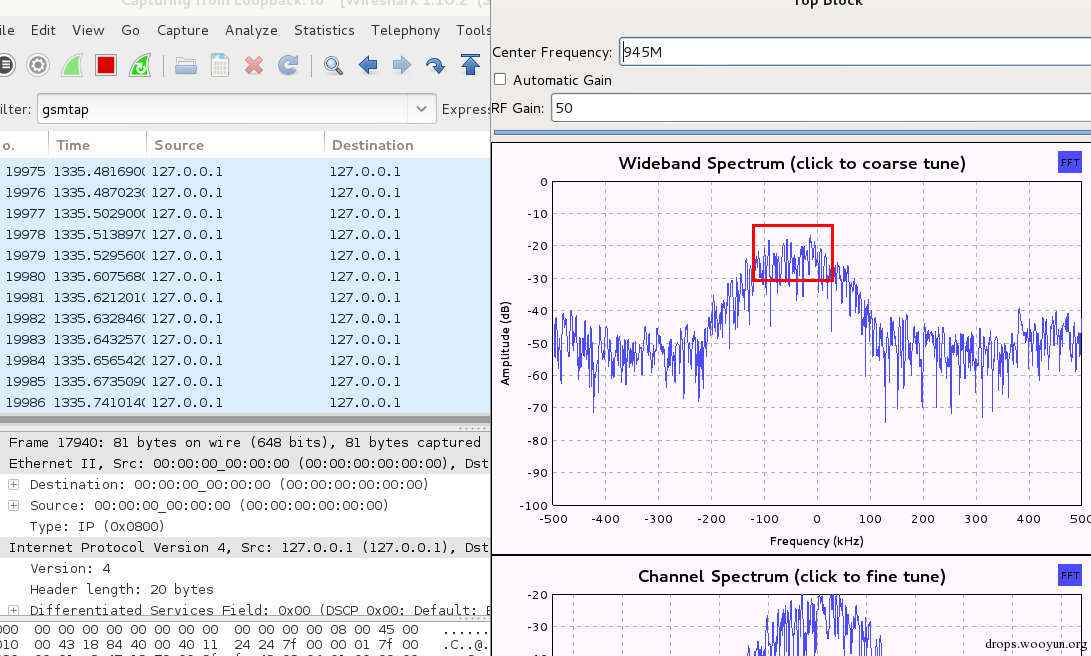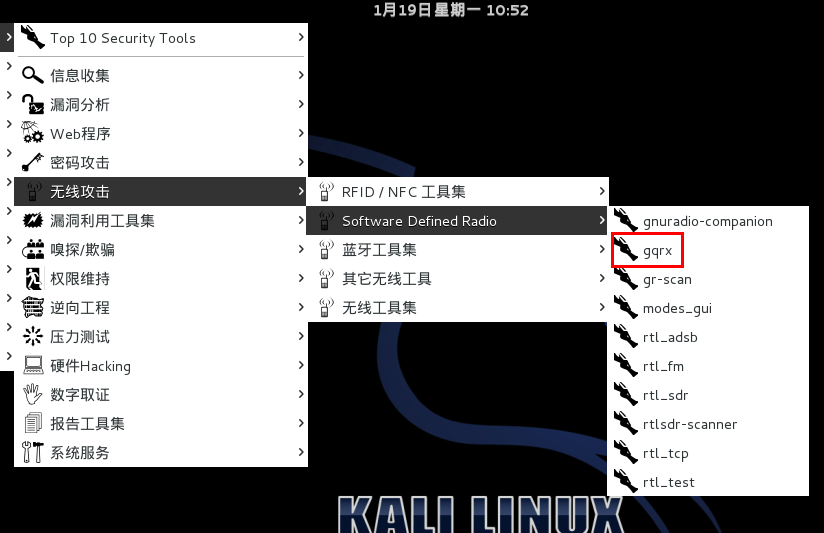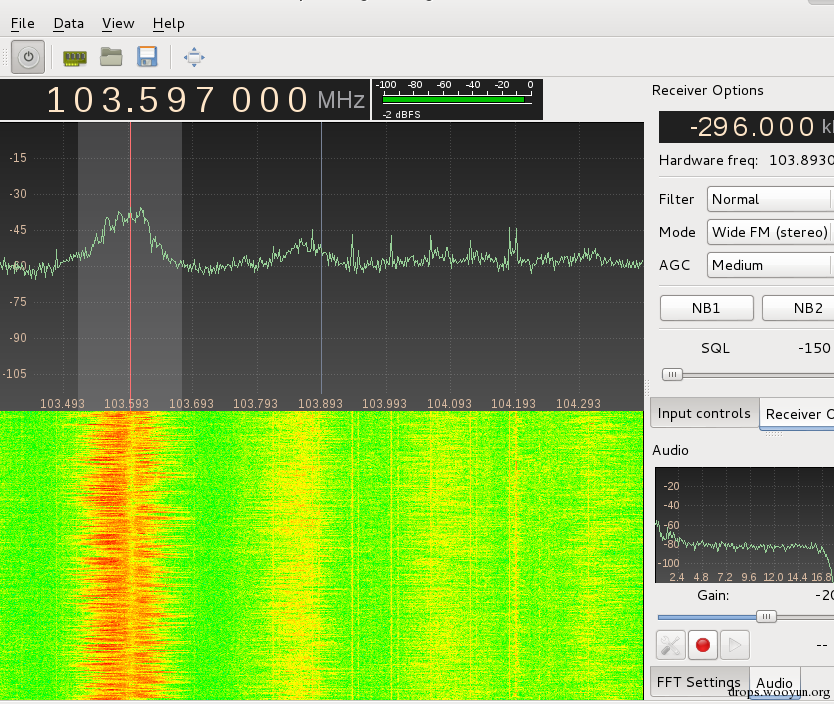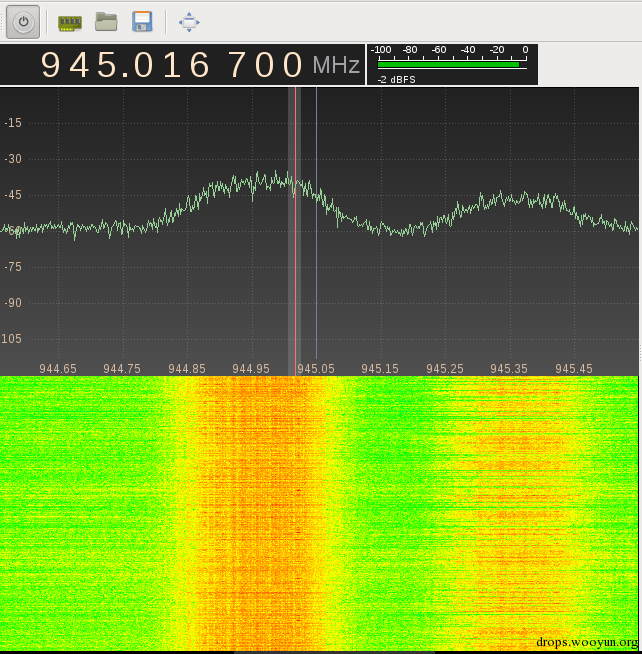PS：听到我耳朵都快怀孕了也只是沙沙声和蜂鸣声，难道有干扰信号？说好的不加密呢 ToT

# 0x05 参考文献

http://sdr.osmocom.org/trac/wiki/rtl-sdr

http://domonkos.tomcsanyi.net/?p=428

RTL-SDR Tutorial: Analyzing GSM with Airprobe/GR-GSM and Wireshark

http://sec.sipsik.net/gsm/baseband/domonkos.tomcsanyi.net/

http://piggybird.net/2014/03/ructf-2014-quals-misc-500-gsm-writeup/

https://lists.srlabs.de/pipermail/a51/2010-July/000688.html

https://srlabs.de/airprobe-how-to/

Cracking and sniffing GSM with a RTL-SDR